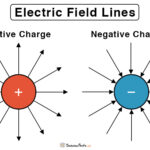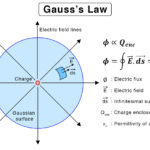Home / Physics / Drag Force

# Drag Force

## What is Drag Force

The resistance offered by a fluid, like air and water, is called the drag force or drag when an object moves through it. It acts in a direction opposite to the object’s motion. It is generated by the relative velocity between the solid object and the fluid. Whether the object or fluid is moving, drag occurs as long as there is a difference in their velocities. Because it is resistant to motion, drag tends to slow down the object. An effective way to reduce it is to alter the shape of the object and make it streamline.

## Examples of Drag Force

There are quite a few examples of the drag force in daily life. Objects experience it when passing through air or water.

• A car driving on a highway
• A ship sailing in the ocean
• A skydiver falling through the sky experiences drag after opening the parachute.
• A swimmer swimming in a pool
• A racing car speeding through a circuit
• An airplane flying through the air

## Drag Force Equation

When the drag takes place in the presence of air, it is known as aerodynamic drag. When it takes place in water, it is known as hydrodynamic drag. The following equation gives the magnitude of the drag force.

FD = ½CρAv2

Where,

C is the drag coefficient, which is dimensionless with values depending upon the shape of the object. (For example, C = 0.47 for a sphere and C = 0.82 for a long cylinder)

ρ is the density of the fluid.

v is the velocity of the object.

A is the projected cross-sectional area of the object in a direction perpendicular to the object’s motion.

Unit of drag force: Newton or N

Dimension of drag force: MLT-2

The above equation applies to almost all fluids. As can be seen, FD is proportional to the square of the velocity, i.e., FD v2. This relationship is quadratic, and hence, the equation is also called quadratic drag force.

The work done by the drag force per unit time, also known as power, is the product of FD times the velocity.

P = FD x v

#### How to Find Terminal Velocity with Drag Force

When an object falls through the air from a high altitude, it experiences both gravity and drag force. Since gravity accelerates the object, its velocity will increase. Consequently, the drag force will increase and be equal in the magnitude of the gravity after some time. When it happens, there is no force acting on the object, which moves through the air with a constant velocity. This velocity is known as terminal velocity vT and is derived as follows.

At terminal velocity, v = vT. Therefore, the drag force is,

FD = ½CρAvT2

And the force due to gravity is,

F = mg

Where,

m is the mass of the object

g is the acceleration due to Earth’s gravity

Equating the two equations,

½CρAvT2 = mg

Or, vT = sqrt(2mg/CρA)

Viscous Drag Force

The equation for FD does not apply in all situations. For viscous fluids, like honey and molasses, the drag force depends on the viscosity η. If the speed v is low (laminar flow), then the drag has a linear relationship with the velocity. For a spherical object of radius R, the magnitude of the drag force is given by Stokes equation.

FD = 6πηRv

This kind of resistance where the liquid’s viscosity plays an important role is called the viscous drag force. Stokes equation is used to study small particles suspended in a liquid.

## Application of Drag Force

Many researchers widely use the drag force to study the motion of vehicles, airplanes, and rockets. These works are carried out in a laboratory where winds are created in an air tunnel. By placing an object, like a model aircraft, measuring and analyzing the drag become possible. These studies enable the researchers to design aircraft that can reduce the drag force.

## Lift and Drag Force

Another physical quantity that is often associated with drag is the lift. Lift is termed as the aerodynamic force that is perpendicular to the object’s motion. As the name suggests, it is responsible for lifting an object from the ground. In an airplane, the lift is created by a difference in air pressure above and below the wings. Its direction is directly opposite to the weight of the plane.

Lift is a mechanical force that is generated by the interaction of an object with a fluid. Therefore, the presence of fluid is essential to generate a lift. This point is easy to understand because, in some planets and moons, where there is no atmosphere, it is practically impossible to lift and fly a plane.

## Drag Forces and Friction

Like drag, friction is also the resistance to an object’s motion. However, the friction takes place at the point of contact between the object and a surface. If the surface is fluid, as in the case of objects moving through a fluid, it is called fluid friction.

Unlike the drag force, the frictional force does not have a velocity dependence. This independence means that the force remains constant even if the object speeds up or slows down.

Article was last reviewed on Saturday, December 5, 2020

### Related articlesElectric Field LinesToroid Magnetic FieldGauss’s LawElectric Flux